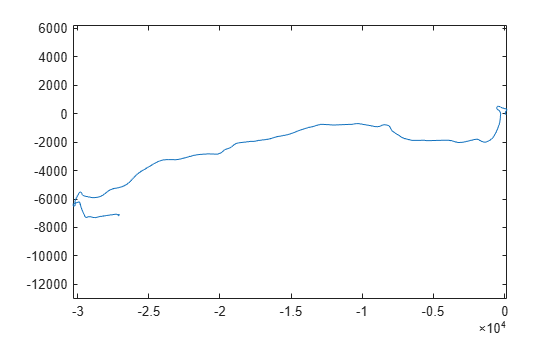Main Content

# latlon2local

Convert geographic coordinates to local Cartesian coordinates

## Syntax

``[xEast,yNorth,zUp] = latlon2local(lat,lon,alt,origin)``

## Description

example

````[xEast,yNorth,zUp] = latlon2local(lat,lon,alt,origin)` converts point locations given by `lat`, `lon`, and `alt` from geographic coordinates to local Cartesian coordinates returned as `xEast`, `yNorth`, and `zUp`. `origin` specifies the anchor of the local coordinate system as a vector of the form [latOrigin,lonOrigin,altOrigin]. Local x, y, z coordinates align with east, north and up directions, respectively. `alt` and `altOrigin` are altitudes as returned by a typical GPS sensor.```

## Examples

collapse all

Load a GPS route.

`d = load('geoRoute.mat');`

Define the origin in geographic coordinates, latitude and longitude.

```alt = 10; % 10 meters is an approximate altitude in Boston, MA origin = [d.latitude(1), d.longitude(1), alt];```

Convert the route from geographic coordinates to Cartesian coordinates, x and y.

`[xEast,yNorth] = latlon2local(d.latitude,d.longitude,alt,origin);`

Plot the route in Cartesian coordinates.

```figure; plot(xEast,yNorth) axis('equal'); % set 1:1 aspect ratio to see real-world shape```## Input Arguments

collapse all

Latitude coordinates, in degrees, specified as a numeric scalar or vector. Value must be in the range [–90, 90]. `lat` must be the same length as `lon`.

Example: `lat = 42.3648`

Data Types: `single` | `double`

Longitude coordinates, in degrees, specified as a numeric scalar or vector. Value must be in the range [–180, 180]. `lon` must be the same length as `lat`.

Example: `lon = -71.0214`

Data Types: `single` | `double`

Altitude, in meters, specified as a numeric scalar or vector.

Example: `10`

Data Types: `single` | `double`

Anchor of local coordinate system, specified as a three-element vector of the form [latOrigin,lonOrigin,altOrigin].

Example: `[42.3648, -71.0214, 10.0];`

Data Types: `single` | `double`

## Output Arguments

collapse all

x-coordinates, returned as a numeric scalar or vector, in meters.

`xEast` is the same class as `lat`. However, if any of the input arguments is of class `single`, then `xEast` is of class `single`.

y-coordinates, returned as a numeric scalar or vector, in meters.

`yNorth` is the same class as `lon`. However, if any of the input arguments is of class `single`, then `yNorth` is of class `single`.

Altitude, returned as a numeric scalar or vector, in meters.

`zUp` is the same class as `alt`. However, if any of the input arguments is of class `single`, then `zUp` is of class `single`.

## Tips

• The latitude and longitude of the geographic coordinate system use the WGS84 standard that is commonly used by GPS receivers.

• This function defines altitude as the height, in meters, above the WGS84 reference ellipsoid.

• Some GPS receivers use standards other than WGS84. Conversions using other ellipsoids are available in the Mapping Toolbox. This function addresses the most common conversion between geographic locations and Cartesian coordinates used by the on-board sensors of a vehicle.

## See Also

Introduced in R2020a

## Support

#### Implementing an Adaptive Cruise Controller with Simulink

Download technical paper